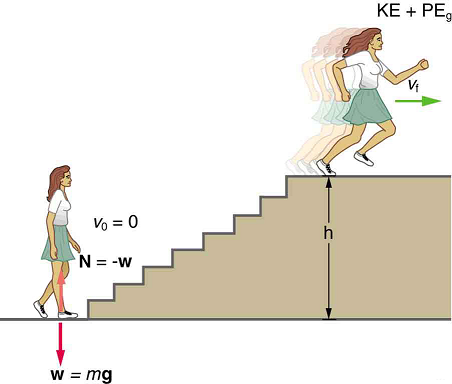$$\require{cancel}$$

# 7.7: Power

•• OpenStax
• General Physics at OpenStax CNX

Learning Objectives

By the end of this section, you will be able to:

• Calculate power by calculating changes in energy over time.
• Examine power consumption and calculations of the cost of energy consumed.

## What is Power?

Power—the word conjures up many images: a professional football player muscling aside his opponent, a dragster roaring away from the starting line, a volcano blowing its lava into the atmosphere, or a rocket blasting off, as in Figure.Figure $$\PageIndex{1}$$: This powerful rocket on the Space Shuttle Endeavor did work and consumed energy at a very high rate. (credit: NASA)

These images of power have in common the rapid performance of work, consistent with the scientific definition of power $$P$$ as the rate at which work is done.

Power

Power is the rate at which work is done.

$P = \dfrac{W}{t}$

The SI unit for power is the watt $$W$$, where 1 watt equals 1 joule/second $$(1 \, W = 1 \, J/s)$$.

Because work is energy transfer, power is also the rate at which energy is expended. A 60-W light bulb, for example, expends 60 J of energy per second. Great power means a large amount of work or energy developed in a short time. For example, when a powerful car accelerates rapidly, it does a large amount of work and consumes a large amount of fuel in a short time.

## Calculating Power from Energy

Example $$\PageIndex{1}$$: Calculating the Power to Climb Stairs

What is the power output for a 60.0-kg woman who runs up a 3.00 m high flight of stairs in 3.50 s, starting from rest but having a final speed of 2.00 m/s? (See Figure.)Figure $$\PageIndex{2}$$:When this woman runs upstairs starting from rest, she converts the chemical energy originally from food into kinetic energy and gravitational potential energy. Her power output depends on how fast she does this.

Strategy and Concept

The work going into mechanical energy is $$W = KE + PE$$. At the bottom of the stairs, we take both $$KE$$ and $$PE$$ as initially zero; thus $$W = KE_f + PE_g = \frac{1}{2}mv_f^2 + mgh,$$ where $$h$$ is the vertical height of the stairs. Because all terms are given, we can calculate $$W$$ and then divide it by time to get power.

Solution

Substituting the expression for $$W$$ into the definition of power given in the previous equation, $$P = W/t$$ yields

$W = \dfrac{W}{t} = \dfrac{\frac{1}{2}mv_f^2 + mgh}{t}.$

Entering known values yields

$P = \dfrac{0.5(60 \, kg)(2.00 m/s^2) + (60.0 \, kg)(9.80 m/s^2)(3.00 \, m)}{3.50 \, s}$

$= \dfrac{120 \, J + 1764 \, J}{3.50 \, s}$

$= 538 \, W.$

Discussion

The woman does 1764 J of work to move up the stairs compared with only 120 J to increase her kinetic energy; thus, most of her power output is required for climbing rather than accelerating.

It is impressive that this woman’s useful power output is slightly less than 1 horsepower $$(1 \, hp = 746 \, W)$$. People can generate more than a horsepower with their leg muscles for short periods of time by rapidly converting available blood sugar and oxygen into work output. (A horse can put out 1 hp for hours on end.) Once oxygen is depleted, power output decreases and the person begins to breathe rapidly to obtain oxygen to metabolize more food—this is known as the aerobic stage of exercise. If the woman climbed the stairs slowly, then her power output would be much less, although the amount of work done would be the same.

Making Connections: Take-Home Investigation

Examples of power are limited only by the imagination, because there are as many types as there are forms of work and energy. (See Table for some examples.) Sunlight reaching Earth’s surface carries a maximum power of about 1.3 kilowatts per square meter $$kW/m^2$$ A tiny fraction of this is retained by Earth over the long term. Our consumption rate of fossil fuels is far greater than the rate at which they are stored, so it is inevitable that they will be depleted. Power implies that energy is transferred, perhaps changing form. It is never possible to change one form completely into another without losing some of it as thermal energy. For example, a 60-W incandescent bulb converts only 5 W of electrical power to light, with 55 W dissipating into thermal energy. Furthermore, the typical electric power plant converts only 35 to 40% of its fuel into electricity. The remainder becomes a huge amount of thermal energy that must be dispersed as heat transfer, as rapidly as it is created. A coal-fired power plant may produce 1000 megawatts; 1 megawatt (MW) is $$10^6$$ of electric power. But the power plant consumes chemical energy at a rate of about 2500 MW, creating heat transfer to the surroundings at a rate of 1500 MW. (See Figure.)